## 1 Introduction

When Arie Hordijk was appointed at the Leiden University in 1976, I became his first PhD student in Leiden. Hordijk was the successor of Guus Zoutendijk, who has chosen to leave the university for a position as chairman of the executive board of the Delta Lloyd Group. Zoutendijk was the supervisor of my master thesis and a leading expert in linear and nonlinear programming. Looking for a PhD project Hordijk suggested linear programming (for short, LP) for the solution of Markov Decision Processes (for short, MDPs). LP for MDPs was introduced by D’Epenoux (1960) for the discounted case. De Ghellinck (1960) as well as Manne (1960) obtained LP formulations for the average reward criterion in the irreducible case. The first analysis of LP for the multichain case was given by Denardo and Fox (1968). Our interest was raised by Derman’s remark (Derman 1970, p. 84): “No satisfactory treatment of the dual program for the multiple class case has been published”.

We started to work on this subject and succeeded to present a satisfactory treatment of the dual program for multichained MDPs. We proved a theorem from which a simple algorithm follows for the determination of an optimal deterministic policy (Hordijk and Kallenberg 1979). In Sect. 3 we describe this approach. Furthermore, we present in Sect. 3 some examples which show the essential difference between irreducible, unichained and multichained MDPs. These examples show for general MDPs:

1. 1.

An extreme optimal solution of the dual program may have in some state more than one positive variable and consequently an extreme feasible solution of the dual program may correspond to a nondeterministic policy (Example 2).

2. 2.

Two different solutions may correspond to the same deterministic policy (Example 3).

3. 3.

An nonoptimal solution of the dual program may correspond to an optimal deterministic policy (Example 4).

4. 4.

The results of the unichain case cannot be generalized to the general single chain case (Example 5).

The second topic of this article concerns additional constraints. Chapter 7 of Derman’s book deals with this subject and has as title “State-action frequencies and problems with constraints”. This chapter may be considered as the starting point for the study of MDPs with additional constraints.

For unichained MDPs with additional constraints, Derman has shown that an optimal policy can be found in the class of stationary policies. We have generalized these results in the sense that for multichained MDPs stationary policies are not sufficient; however, in that case there exists an optimal policy in the class of Markov policies. This subject is presented in Sect. 4.

Derman’s book also deals with some applications, for instance optimal stopping and replacement problems. In the last part, Sect. 5, of this paper we will discuss LP methods for the following applications:

1. 1.

Optimal stopping problems.

2. 2.

Replacement problems:

1. (a)

General replacement problems;

2. (b)

Replacement problems with increasing deterioration;

3. (c)

4. (d)

Separable replacement problems.

3. 3.

Multi-armed bandit problems.

4. 4.

Separable problems with both the discounted and the average reward criterion.

## 2 Notations and definitions

Let S be the finite state space and A(i) the finite action set in state iS. If in state i action aA(i) is chosen, then a reward r i (a) is earned and p ij (a) is the transition probability that the next state is state j.

A policy R is a sequence of decision rules: R=(π 1,π 2,…,π t,…), where π t is the decision rule at time point t, t=1,2,…. The decision rule π t at time point t may depend on all available information on the system until time t, i.e., on the states at the time points 1,2,…,t and the actions at the time points 1,2,…,t−1.

Let C denote the set of all policies. A policy is said to be memoryless if the decision rules π t are independent of the history; it depends only on the state at time t. We call C(M) the set of the memoryless policies. Memoryless policies are also called Markov policies.

If a policy is memoryless and the decision rules are independent of the time point t, then the policy is called stationary. Hence, a stationary policy is determined by a nonnegative function π on S×A, where S×A={(i,a)∣iS, aA(i)}, such that ∑ a π ia =1 for every iS. The stationary policy R=(π,π,…) is denoted by π . The set of stationary policies is notated by C(S).

If the decision rule π of a stationary policy is nonrandomized, i.e., for every iS, we have π ia =1 for exactly one action a, then the policy is called deterministic. A deterministic policy can be described by a function f on S, where f(i) is the chosen action in state i. A deterministic policy is denoted by f and the set of deterministic policies by C(D).

A matrix P=(p ij ) is a transition matrix if p ij ≥0 for all (i,j) and ∑ j p ij =1 for all i. Notice that P is a stationary Markov chain. For a Markov policy R=(π 1,π 2,…) the transition matrix P(π t) is defined by

$$\{P(\pi^t)\}_{ij} = \sum_a p_{ij}(a) \pi^t_{ia}$$

and the vector r(π t), defined by

$$\{r(\pi^t)\}_i = \sum_a r_i(a)\pi^t_{ia},$$

is called the reward vector.

Let the random variables X t and Y t denote the state and action at time t. Given starting state i, policy R and a discount factor α∈(0,1), the discounted reward and the average reward are denoted by $$v^{\alpha}_{i}(R)$$ and ϕ i (R), respectively, and defined by

$$v^\alpha_i(R) = \sum_{t=1}^\infty \alpha^{t-1} \mathbb{E}_{i,R} \{r_{X_t}(Y_t)\}$$

and

$$\phi_i(R) = \liminf_{T\rightarrow\infty} \frac{1}{T} \sum_{t=1}^T \mathbb{E}_{i,R}\{r_{X_t}(Y_t)\},$$

respectively.

The value vectors v α and ϕ for discounted and average rewards are defined by $$v^{\alpha}_{i} = \sup_{R}v^{\alpha}_{i}(R)$$, iS, and ϕ i =sup R ϕ i (R), iS, respectively.

A policy R is a discounted optimal policy if $$v^{\alpha}_{i}(R^{*})= v^{\alpha}_{i}$$, iS; similarly, R is an average optimal policy if ϕ i (R )=ϕ i , iS. It is well known that, for both discounted as average rewards, an optimal policy exists and can be found within C(D), the class of deterministic policies.

An MDP is called irreducible if, for all deterministic decision rules f, in the Markov chain P(f) all states belong to a single ergodic class.

An MDP is called unichained if, for all deterministic decision rules f, in the Markov chain P(f) all states belong to a single ergodic class plus a (perhaps empty and decision rule dependent) set of transient states. In the weak unichain case every optimal deterministic policy f has a unichain Markov chain P(f); in the general single chain case at least one optimal deterministic policy f has a unichain Markov chain P(f);

An MDP is called multichained if there may be several ergodic classes and some transient states; these classes may vary from policy to policy.

An MDP is communicating if for every i,jS there exists a deterministic policy f , which may depend on i and j, such that in the Markov chain P(f) state j is accessible from state i.

It is well known that for irreducible, unichained and communicating MDPs the value vector has identical components. Hence, in these cases one uses, instead of a vector, a scalar ϕ for the value.

## 3 LP for MDPs with the average reward criterion

### 3.1 The irreducible case

In Chap. 6, pp. 78–80, of Derman’s book the following result can be found, which originates from Manne (1960).

### Theorem 1

Let (v ,u ) and x be optimal solutions of (1) and (2), respectively, where

$$\min\biggl\{v \mid v + \sum_j \{\delta_{ij} - p_{ij}(a)\}u_j \geq r_i(a),(i,a)\in S \times A\biggr\}$$
(1)

and

$$\max\left\{\sum_{i,a} r_i(a)x_i(a) \left\vert \begin{array}{l}\sum_{i,a} \{\delta_{ij} - p_{ij}(a)\}x_i(a) = 0,\quad j \in S \\\sum_{i,a} x_i(a) = 1 \\x_i(a) \geq0,\quad i \in S, a \in A(i)\end{array}\right. \right\}.$$
(2)

Let $$f_{*}^{\infty}$$ be such that $$x_{i}^{*}(f_{*}(i)) > 0$$, iS. Then, $$f_{*}^{\infty}$$ is well defined and an average optimal policy. Furthermore, v =ϕ, the value.

### Theorem 2

Let (v ,u ) and x be optimal solutions of (1) and (2), respectively. Let $$S_{*} = \{i\mid\sum_{a} x^{*}_{i}(a) >0\}$$. Choose $$f_{*}^{\infty}$$ such that $$x_{i}^{*}(f_{*}(i)) > 0$$ if iS and choose f (i) arbitrarily if iS . Then, $$f_{*}^{\infty}$$ is an average optimal policy. Furthermore, v =ϕ, the value.

This linear programming result for unichained MDPs was derived by Denardo (1970). I suppose that Derman was also aware of this result, although it was not explicitly mentioned in his book. Theorem 2 on p. 75 and the subsequent text on p. 76 are the reason of my supposition. The result of Theorem 2, but with a different proof, is part of my thesis (Kallenberg 1980), which was also published in Kallenberg (1983).

### 3.3 The communicating case

Since the value vector ϕ is constant in communicating MDPs, the value ϕ is the unique v -part of an optimal solution (v ,u ) of the linear program (1). One would expect that an optimal policy could also be obtained from the dual program (2). The next example shows that—in contrast with the irreducible and the unichain case—in the communicating case the optimal solution of the dual program doesn’t provide an optimal policy, in general.

### Example 1

S={1,2,3}; A(1)={1,2}, A(2)={1,2,3}, A(3)={1,2}. r 1(1)=0, r 1(2)=2; r 2(1)=1, r 2(2)=1, r 2(3)=3; r 3(1)=2; r 3(2)=4. p 12(1)=p 11(2)=p 23(1)=p 21(2)=p 22(3)=p 32(1)=p 33(2)=1 (other transitions are 0). This is a multichain and communicating model. The value is 4 and $$f_{*}^{\infty}$$ with f (1)=f (2)=1, f (3)=2 is the unique optimal deterministic policy.

The primal linear program (1) becomes for this model

\min\left\{v \,\left|\,\begin{array}{l}v + u_1 - u_2 \geq0; v \geq2; v + u_2 - u_3 \geq1; v - u_1 + u_2\geq1\\\noalign{\vspace{3pt}}v \geq3; v - u_2 + u_3 \geq2; v \geq4\end{array}\right. \right\}

with optimal solution v =4; $$u^{*}_{1} = 0$$, $$u^{*}_{2} = 3$$, $$u^{*}_{3} = 5$$ (v is unique; u is not unique).

The dual linear program is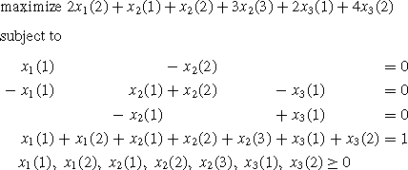For the optimal solution x , we obtain: $$x^{*}_{1}(1) = x^{*}_{1}(2) =x^{*}_{2}(1) = x^{*}_{2}(2) = x^{*}_{2}(3) = x^{*}_{3}(1) = 0;~x^{*}_{3}(2) = 1$$ (this solution is unique).

Proceeding as if this were a unichain model, we choose arbitrary actions in the states 1 and 2. Clearly, this approach may generate a nonoptimal policy.

So, we are not able—in general—to derive an optimal policy from the dual program (2). However, it is possible to find an optimal policy with some additional work. In Example 1 we have seen that the optimal solution x provides an optimal action in state 3, which is the only state of $$S_{*} = \{i\mid\sum_{a} x^{*}_{i}(a) > 0\}$$. The next theorem shows that the states of S always provide optimal actions. For the proof we refer to Kallenberg (2010).

### Theorem 3

Let x be an extreme optimal solution of (2). Take any policy $$f_{*}^{\infty}$$ such that $$x^{*}_{i}(f_{*}(i)) > 0$$, iS . Then, $$\phi_{j}(f_{*}^{\infty}) = \phi$$, jS .

Note that $$S_{*} \not= \emptyset$$ (because $$\sum_{i,a} x^{*}_{i}(a) = 1$$) and that we can find, by Theorem 3, optimal actions f (i) for all iS . Furthermore, one can easily show that S is closed in the Markov chain P(f ).

Since we have a communicating MDP, one can find for each iS an action f (i) such that in the Markov chain P(f ) the set S is reached from state i with a strictly positive probability after one or more transitions. So, the set S\S is transient in the Markov chain P(f ). Therefore, the following search procedure provides the remaining optimal actions for the states S\S .

### Search procedure

1. 1.

If S =S: stop;

Otherwise go to step 2.

2. 2.

Pick a triple (i,a,j) with iS\S , aA(i), jS and p ij (a)>0.

3. 3.

f (i):=a, S :=S ∪{i} and go to step 1.

A second way to find an optimal policy for communicating MDPs is based on the following theorem which is due to Filar and Schultz (1988).

### Theorem 4

An MDP is communicating if and only if for every b∈ℝ|S| such that j b j =0 there exists a $$y \in{\mathbb{R}}_{+}^{|S \times A|}$$ such that i,a {δ ij p ij (a)}y i (a)=b j for all jS.

The following procedure also yields an optimal deterministic policy. This is based on results for multichained MDPs which are discussed in Sect. 3.4.

### Determination y-variables

1. 1.

Choose β∈ℝ|S| such that β j >0, jS and ∑ j β j =1.

2. 2.

Let $$b_{j} = \beta_{j} - \sum_{a} x^{*}_{j}(a)$$, jS.

3. 3.

Determine $$y^{*} \in{\mathbb{R}}_{+}^{|S \times A|}$$ such that $$\sum_{i,a}\{\delta_{ij} - p_{ij}(a)\}y^{*}_{i}(a) = b_{j}$$, jS.

4. 4.

Choose f (i) such that $$y^{*}_{i}(f_{*}(i)) > 0$$ for all iS\S .

### Example 1

(continued)

• Search procedure:

• S ={3}.

• i=2; a=1; j=3; f (2)=1; S ={2,3}.

• i=1; a=1; j=2; f (1)=1; S ={1,2,3}.

• Determination y-variables:

• Choose $$\beta_{1} = \beta_{2} = \beta_{3} = \frac{1}{3}$$.

• Let $$b_{1} = \frac{1}{3}$$, $$b_{2} = \frac{1}{3}$$, $$b_{3} = -\frac{2}{3}$$.

• The system ∑ i,a {δ ij p ij (a)}y i (a)=b j , jS becomes:

$$\begin{array}{lllllllllr}& y_1(1) & & & - & y_2(2) & & & = & \frac{1}{3} \\[0.12cm]- & y_1(1) & + & y_2(1) & + & y_2(2) & - & y_3(1) & = & \frac{1}{3} \\[0.12cm]& & - & y_2(1) & & & + & y_3(1) & = & -\frac{2}{3} \\[0.12cm]\end{array}$$

with a nonnegative solution $$y^{*}_{1}(1) = \frac{1}{3}$$, $$y^{*}_{2}(1) =\frac{2}{3}$$, $$y^{*}_{2}(2) = y^{*}_{3}(1) = 0$$ (this solution is not unique). Choose f (1)=f (2)=1.

### Remarks

1. 1.

The verification of an irreducible or communicating MDP is computationally easy (see Kallenberg 2002); generally, the verification of a unichain MDP is $$\mathcal{NP}$$-complete as shown by Tsitsiklis (2007).

2. 2.

It turns out that the approach with the search procedure can also be used for the weak unichain case.

### 3.4 The multichain case

For multichained MDPs the programs (1) and (2) are not sufficient. For general MDPs the following dual pair of linear programs were proposed by Denardo and Fox (1968):

$$\min\left\{\sum_j \beta_j v_j \left\vert \begin{array}{l@{\quad}l}\sum_j \{ \delta_{ij} - p_{ij}(a) \}v_j \geq0,& (i,a) \in S \times A\\[0.1cm]v_i + \sum_j \{ \delta_{ij} - p_{ij}(a) \}u_j \geq r_i(a) ,& (i,a)\in S \times A\end{array}\right\} \right.$$
(3)

and

$$\max\left\{\sum_{(i,a)} r_i(a) x_i(a) \left\vert \begin{array}{l@{\quad}l}\sum_{i,a} \{\delta_{ij} - p_{ij}(a)\}x_i(a) = 0, & j \in S \\ [0.1cm]\sum_a x_j(a) + \sum_{i,a} \{\delta_{ij} - p_{ij}(a)\}y_i(a) = \beta _j, & j \in S \\ [0.1cm]x_i(a),y_i(a) \geq0,\quad i \in S, a \in A(i)\end{array}\right\}, \right.$$
(4)

where β j >0 for all jS.

In Denardo and Fox (1968) it was shown that if (v ,u ) is an optimal solution of the primal problem (3), than v =ϕ, the value vector.

Notice that if the value vector ϕ is constant, i.e., ϕ has identical components, then $$\sum_{j} \{ \delta_{ij} - p_{ij}(a)\}v^{*}_{j} = \sum_{j} \{ \delta_{ij} - p_{ij}(a) \}\phi= \{1 - 1\}\phi = 0$$. Hence, the first set of inequalities of (3) is superfluous and (3) can be simplified to (1) with as dual program (2).

Furthermore, Denardo and Fox have derived the following result (see pp. 73–75 in Derman 1970).

### Lemma 1

Let $$f_{*}^{\infty}\in C(D)$$ be an optimal policy and let (v =ϕ,u ) be an optimal solution of the primal program (3). Then,

$$\left\{\begin{array}{l@{\quad}l}\sum_j \{ \delta_{ij} - p_{ij}(f_*) \}\phi_j = 0, & i \in S \\[0.1cm]\phi_i + \sum_j \{ \delta_{ij} - p_{ij}(f_*) \}u^*_j = r_i(f_*) , &i \in R(f_*)\end{array}\right.$$

where R(f )={ii is recurrent in the Markov chain P(f )}.

Lemma 1 asserts that in any optimal solution of the primal program (3) one can always select actions f (i) such that ∑ j {δ ij p ij (f )}ϕ j =0, iS, and $$\phi_{i} + \sum_{j} \{ \delta_{ij} - p_{ij}(f_{*}) \}u^{*}_{j} =r_{i}(f_{*})$$ for all i in a nonempty subset S(f ) of S. Furthermore, the following result holds, given such policy $$f_{*}^{\infty}$$ and a companion S(f ) (see pp. 75–76 in Derman 1970).

### Lemma 2

If all states of S\S(f ) are transient in the Markov chain P(f ), then policy $$f_{*}^{\infty}$$ is an average optimal policy.

If we are fortunate in our selection of $$f_{*}^{\infty}$$, then the states of S\S(f ) are transient in the Markov chain P(f ) and policy $$f_{*}^{\infty}$$ is an average optimal policy. However, we may not be so fortunate in our selection of $$f_{*}^{\infty}$$. In that case, Derman suggests the following approach to find an optimal policy (see pp. 76–78 in Derman’s book 1970). Let S 1 be defined by

$$S_1 = \left\{i \,\left| \,\exists a \in A(i)\ \mbox{such that}\ \left\{\begin{array}{l}\sum_j \{ \delta_{ij} - p_{ij}(a) \}v_j = 0 \\[0.1cm]v_i + \sum_j \{ \delta_{ij} - p_{ij}(a) \}u_j = r_i(a)\end{array}\right.\right\}.\right.$$
(5)

By Lemma 1, S\S 1 must consist entirely of transient states under every optimal policy. Let S 2 be defined by

$$S_2 = \left\{i \in S_1 \,\left\vert\,\begin{array}{l}\exists a \in A(i)\ \mbox{with}\ \left\{\begin{array}{l}\sum_j \{ \delta_{ij} - p_{ij}(a) \}v_j = 0 \\[0.1cm]v_i + \sum_j \{ \delta_{ij} - p_{ij}(a) \}u_j = r_i(a)\end{array}\right. \\ [0.4cm]\mbox{which satisfies}\ p_{ij}(a) = 0\ \mbox{for all}\ j \in S\backslash S_1\end{array}\right\}. \right.$$
(6)

Also by Lemma 1, the states of S 1\S 2 must be transient under at least one optimal policy $$f_{*}^{\infty}$$. Let S 3 and A 3(i), iS 3 be defined as

$$S_3 = S \backslash S_2; \qquad A_3(i) = \biggl\{a \in A(i)\,\bigg {|}\,\sum_j \{ \delta_{ij} - p_{ij}(a) \}\phi_j = 0\biggr\},\quad i \in S_3.$$
(7)

Consider the following linear program

$$\min\biggl\{\sum_{j \in S_3} w_j \,\bigg{|}\,\sum_{j \in S_3} \{\delta_{ij} - p_{ij}(a)\}w_j \geq s_i(a),i \in S_3,\ a \in A_3(i)\biggr\},$$
(8)

where $$s_{i}(a) = r_{i}(a) - \sum_{j \notin S_{3}} \{ \delta_{ij} -p_{ij}(a) \}u_{j}^{*} - \phi_{i}$$.

### Theorem 5

1. (1)

The linear program (8) has a finite optimal solution.

2. (2)

Let w be an optimal solution of (8). Then, for each iS 3 there exists at least one action f (i) satisfying $$\sum_{j \in S_{3}} \{\delta_{ij} -p_{ij}(f_{*})\}w^{*}_{j} = s_{i}(f_{*})$$.

3. (3)

Let $$f_{*}^{\infty}$$ be such that

$$\left\{\begin{array}{l@{\quad}l}\sum_j \{ \delta_{ij} - p_{ij}(f_*) \}\phi_j = 0 ,&i \in S_2\\\phi_i + \sum_j \{ \delta_{ij} - p_{ij}(f_*) \}u^*_j = r_i(f_*) ,&i\in S_2\end{array}\right.$$

and $$\sum_{j \in S_{3}} \{\delta_{ij} - p_{ij}(f_{*})\}w^{*}_{j} =s_{i}(f_{*})$$, iS 3. Then, $$f_{*}^{\infty}$$ is an average optimal policy.

Hence, in order to find an optimal policy in the multichain case, by the results of Denardo and Fox (1968) and Derman (1970), one has to execute the following procedure:

1. 1.

Determine an optimal solution (v ,u ) of the linear program (3) to find the value vector ϕ=v .

2. 2.

Determine, by (5), (6) and (7), the sets S 1,S 2,S 3 and A 3(i), iS 3.

3. 3.

Compute $$s_{i}(a) = r_{i}(a) - \sum_{j \notin S_{3}} \{\delta_{ij} - p_{ij}(a) \}u_{j}^{*} - \phi_{i}$$, iS 3, aA 3(i).

4. 4.

Determine an optimal solution w of the linear program (8).

5. 5.

Determine an optimal policy $$f_{*}^{\infty}$$ as described in Theorem 5.

This rather complicated approach elicited from Derman the remark (see Derman 1970, p. 84): “No satisfactory treatment of the dual program for the multiple class case has been published”, which was for Hordijk and myself the reason to start research on this topic. In Hordijk and Kallenberg (1979) the following result was proved.

### Theorem 6

Let (x ,y ) be an extreme optimal solution of the dual program (4). Then, any stationary deterministic policy $$f_{*}^{\infty}$$ such that

$$\left\{\begin{array}{l@{\quad}l}x^*_i(f_*(i)) > 0 & \mbox{\textit{if}}\ i \in S_* \\y^*_i(f_*(i)) > 0 & \mbox{\textit{if}}\ i \notin S_*\end{array}\right.,\quad\mbox{where}\ S_* = \biggl\{i\mid\sum_a x^*_i(a) > 0\biggr\},$$

is well-defined and is an average optimal policy.

This result is based on the following propositions, where:

• Proposition 1 is related to Lemma 1;

• Proposition 2 is related to the definitions of S 2;

• Proposition 3 is related to Lemma 2; it also uses the property that the columns of positive variables of an extreme optimal solution are linearly independent.

### Proposition 1

Let (v =ϕ,u ) be an optimal solution of program (3). Then,

$$\left\{\begin{array}{l@{\quad}l}\sum_j \{ \delta_{ij} - p_{ij}(f_*) \}\phi_j = 0 ,&i \in S\\[0.1cm]\phi_i + \sum_j \{ \delta_{ij} - p_{ij}(f_*) \}u^*_j = r_i(f_*) ,&i\in S_*.\end{array}\right.$$

### Proposition 2

The subset S of S is closed in the Markov chain P(f ).

### Proposition 3

The states of S\S are transient in the Markov chain P(f ).

The correspondence between feasible solutions (x,y) of (4) and randomized stationary policies π is given by the following mappings. For a feasible solution (x,y) the corresponding policy π (x,y) is defined by

$$\pi_{ia}(x,y) = \left\{\begin{array}{l@{\quad}l}\frac{x_i(a)}{\sum_a x_i(a)} & \mbox{if} \ \sum_a x_i(a) > 0 \\ [0.2cm]\frac{y_i(a)}{\sum_a y_i(a)} & \mbox{if} \ \sum_a x_i(a) = 0.\end{array}\right.$$
(9)

Conversely, for a stationary policy π , we define a feasible solution (x π,y π) of the dual program (4) by

$$\left\{\begin{array}{l}x^\pi_i(a) = \{\sum_j \beta_j\{P^*(\pi)\}_{ji} \} \cdot\pi_i(a)\\ [0.1cm]y^\pi_i(a) = \{\sum_j \beta_j\{D(\pi)\}_{ji} + \sum_j\gamma_j\{P^*(\pi)\}_{ji} \} \cdot\pi_i(a),\end{array}\right.$$
(10)

where P (π) and D(π) are the stationary and the deviation matrix of the transition matrix P(π); γ j =0 on the transient states and constant on each recurrent class under P(π) (for the precise definition of γ see Hordijk and Kallenberg 1979).

Now, we will present some examples which show the essential difference between irreducible, unichained and multichained MDPs.

### Example 2

It is well-known that in the irreducible case each extreme optimal solution has exactly one positive x-variable. It is also well known that in other cases some states can have no positive x-variables, i.e., S is a proper subset of S.

This example shows an MDP with an extreme optimal solution which has two positive x-variables for some state. Hence, the two corresponding deterministic policies, which can constructed via Theorem 6, are both optimal.

Furthermore, this extreme feasible solution is mapped on a nondeterministic policy. Let S={1,2,3}; A(1)={1}, A(2)={1}, A(3)={1,2}; r 1(1)=1, r 2(1)=2, r 3(1)=4, r 3(2)=3; p 13(1)=p 23(1)=p 31(1)=p 32(2)=1 (other transitions are 0).

The dual program (4) of this MDP is (take $$\beta_{1} =\beta_{2} = \frac{1}{4}, \beta_{3} = \frac{1}{2}$$):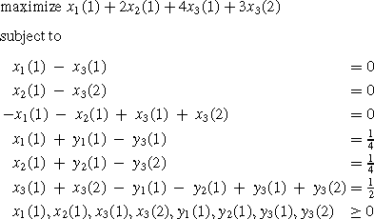The feasible solution (x,y), where $$x_{1}(1) = x_{2}(1) = x_{3}(1) =x_{3}(2) = \frac{1}{4}$$, y 1(1)=y 2(1)=y 3(1)=y 3(2)=0, is an extreme optimal solution. Observe that state 3 has two positive x-variables.

### Example 3

This example shows that the mapping (9) is not a bijective mapping. Let S={1,2,3,4}; A(1)={1}, A(2)={1,2}, A(3)={1,2}, A(4)={1}; p 12(1)=p 23(1)=p 24(2)=p 33(1)=p 31(2)=p 44(1)=1 (other transitions are 0). Since the rewards are not important for this property, we have omitted these numbers.

The constraints of the dual program are (take $$\beta_{j} = \frac{1}{4}$$, 1≤j≤4):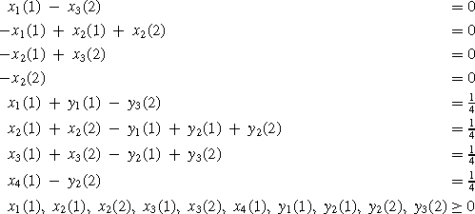First, consider the feasible solution (x 1,y 1) with $$x^{1}_{1}(1) =x^{1}_{2}(1) = \frac{1}{4}$$, $$x^{1}_{2}(2) = x^{1}_{3}(1) = 0$$, $$x^{1}_{3}(2) = x^{1}_{4}(1) = \frac{1}{4}$$; $$y^{1}_{1}(1) = y^{1}_{2}(1) = y^{1}_{2}(2) = y^{1}_{3}(2) = 0$$. This feasible solution is mapped on the deterministic policy $$f_{1}^{\infty}$$ with f 1(1)=f 1(2)=1, f 1(3)=2, f 1(4)=1.

Then, consider the feasible solution (x 2,y 2) with $$x^{2}_{1}(1) =x^{2}_{2}(1) = \frac{1}{6}$$, $$x^{2}_{2}(1) = x^{2}_{3}(1) = 0$$, $$x^{2}_{3}(2) = \frac{1}{6}$$, $$x^{2}_{4}(1)= \frac{1}{2}$$, $$y^{2}_{1}(1) = \frac{1}{6}$$, $$y^{2}_{2}(1) = 0$$, $$y^{2}_{2}(2) =\frac{1}{4}$$, $$y^{2}_{3}(2) = \frac{1}{12}$$. This feasible solution is mapped on the deterministic policy $$f_{2}^{\infty}$$ with f 2(1)=f 2(2)=1, f 2(3)=2, f 2(4)=1. Notice that $$(x^{1},y^{1}) \not= (x^{2},y^{2})$$ and $$f_{1}^{\infty}= f_{2}^{\infty}$$.

### Example 4

This example shows that a feasible nonoptimal solution can be mapped on an optimal policy. Let S={1,2,3}; A(1)=A(2)={1,2}, A(3)={1}; p 12(1)=p 13(2)=p 21(1)=p 22(2)=p 33(1)=1 (other transitions are 0); r 1(1)=1, r 1(2)=r 2(1)=r 2(2)=r 3(1)=0.

The dual program for this model is (take $$\beta_{1} = \beta_{2} = \beta _{3} =\frac{1}{3}$$):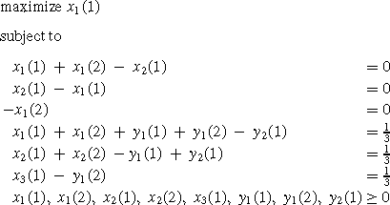The solution (x,y) given by $$x_{1}(1) = \frac{1}{6}$$, x 1(2)=0, $$x_{2}(1) = \frac{1}{6}$$, x 2(2)=0, $$x_{3}(1) = \frac{2}{3}$$, y 1(1)=0, $$y_{1}(2) = \frac{1}{3}$$, $$y_{2}(1) = \frac{1}{6}$$ is a feasible solution, but not an optimal solution. Notice that $$x^{*}_{1}(1) = x^{*}_{2}(1)= x^{*}_{3}(1) = \frac{1}{3}$$ and all other variables 0 is an optimal solution and that the x-part of the optimal solution is unique. However, the policy f which corresponds to (x,y) has f(1)=f(2)=f(3)=1 and is an optimal policy.

### Example 5

In this last example, we show that the general unichain case needs an approach different from the unichain case; even the additional search procedure is not sufficient. In the general unichain case the value vector is a constant vector and the linear programs (1) and (2) may be considered. Let S={1,2,3}; A(1)={1}, A(2)=A(3)={1,2}; r 1(1)=r 2(1)=0, r 2(2)=r 3(1)=1, r 3(2)=0; p 12(1)=p 21(1)=p 22(2)=p 33(1)=p 32(2)=1 (other transitions are 0). This is a general unichained MDP, because the policy f with f(1)=1, f(2)=f (3)=2 is an optimal policy and has a single chain structure. The dual program (2) of this model is: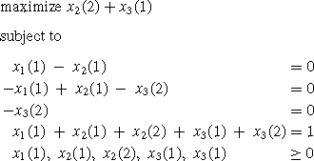x given by x 1(1)=x 2(1)=x 2(2)=x 3(2)=0, x 3(1)=1 is an extreme optimal solution. In state 3, the policy corresponding to x chooses action 1. The choice in state 2 for an optimal policy has to be action 2. Since the set of the states 1 and 2 is closed under any policy, it is impossible to search for actions in these states with transitions to state 3.

## 4 State-action frequencies and problems with constraints

### 4.1 Introduction

“State-action frequencies and problems with constraints” is the title of chapter 7 of Derman’s book. This chapter may be concerned as the starting point for the study of MDPs with additional constraints. In such problems it is not obvious that optimal policies exist. It is also not necessarily true that optimal policies, if they exist, belong to the class C(D) or C(S).

MDPs with additional constraints occur in a natural way in all kind of applications. For instance in inventory management, where one wants to minimize the total costs under the constraint that the shortage is bounded by a given number.

In general, for MDPs with additional constraints, a policy which is optimal simultaneously for all starting states does not exist. Therefore, we consider problems with a given initial distribution β, i.e., β j is a given probability that state j is the starting state. A special case is β j =1 for j=i and β j =0 for $$j \not= i$$, i.e., that state i is the (fixed) starting state.

In many cases reward and cost functions are specified in terms of expectations of some function of the state-action frequencies. Given the initial distribution β, we define for any policy R, any time point t and any state-action pair (i,a)∈S×A, the action-state frequency $$x_{ia}^{R}(t)$$ by

$$x_{ia}^R(t) = \sum_{j \in S} \beta_j \cdot\mathbb{P}_R\{X_t = i,Y_t =a\mid X_1 = j\}.$$
(11)

For the additional constraints we assume that, besides the immediate rewards r i (a), there are also certain immediate costs $$c^{k}_{i}(a)$$, iS, aA(i) for k=1,2,…,m.

Let β be an arbitrary initial distribution. For any policy R, let the average reward and the k-th average cost function with respect to the initial distribution β be defined by

$$\phi(\beta,R) = \liminf_{T \rightarrow\infty}\frac{1}{T}\sum_{t=1}^T \sum_{j \in S}\beta_j \cdot\sum_{i,a} \mathbb{P}_R\{X_t = i, Y_t = a\mid X_1 = j\}\cdot r_i(a)$$
(12)

and

$$c^k(\beta,R)= \liminf_{T \rightarrow\infty} \frac{1}{T}\sum _{t=1}^T \sum_{j \in S}\beta_j \cdot\sum_{i,a} \mathbb{P}_R\{X_t = i, Y_t = a\mid X_1 = j\}\cdot c^k_i(a).$$
(13)

A policy R is a feasible policy for a constrained Markov decision problem, shortly CMDP, if the k-the cost function is bounded by a given number b k for k=1,2,…,m, i.e., if c k(β,R)≤b k , k=1,2,…,m.

An optimal policy R for this criterion is a feasible policy that maximizes ϕ(β,R), i.e.,

$$\phi(\beta,R^*) = \sup_R \{\phi(\beta,R)\mid c^k(\beta,R) \leq b_k, k= 1,2,\dots,m\}.$$
(14)

For any policy R and any T∈ℕ, we denote the average expected state-action frequencies in the first T periods by

$$x^T_{ia}(R) = \frac{1}{T}\sum_{t=1}^T x_{ia}^R(t),\quad(i,a) \in S\times A.$$
(15)

By X(R) we denote the limit points of the vectors {x T(R), T=1,2,…}. For any T∈ℕ, x T(R) satisfies $$\sum_{(i,a)}x_{ia}^{T}(R) =1$$; so also ∑(i,a) x ia (R)=1 for all x(R)∈X(R).

Since $$\mathbb{P}_{\pi^{\infty}}\{X_{t} = i, Y_{t} = a \mid X_{1} = j\} =\{P^{t-1}(\pi)\}_{ji} \cdot\pi_{ia}$$, (i,a)∈S×A for all π C(S), we have $$\lim_{T \rightarrow\infty}x_{ia}^{T}(\pi^{\infty})=\sum_{j \in S} \beta_{j} \{P^{*}(\pi)\}_{ji}\cdot \pi_{ia}$$, i.e., X(π ) consists of only one element, namely the vector x(π), where x ia (π)={β T P (π)} i π ia , (i,a)∈S×A.

Let the policy set C 1 be the set of convergent policies, defined by

$$C_1 = \{R\mid X(R)\ \mbox{consists of one element}\}.$$
(16)

Hence, C(S)⊆C 1. Furthermore, define the vector sets L, L(M), L(C), L(S) and L(D) by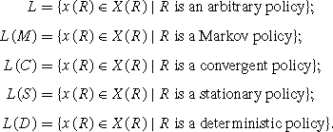The following result is due to Derman (1970, pp. 93–94).

### Theorem 7

$$L = L(M) = \overline{L(S)} = \overline{L(D)}$$, where $$\overline{L(S)}$$ and $$\overline{L(D)}$$ are the closed convex hull of the sets L(S) and L(D), respectively.

### 4.2 The unichain case

Derman has also shown (Derman 1970, pp. 95–96) that in the unichain case a feasible CMDP has an optimal stationary policy. He showed that L(S)=X, where

$$X = \left\{x \in{\mathbb{R}}^{|S \times A|}\left\vert \begin{array}{l@{\quad}l}\sum_{i,a} \{\delta_{ij} - p_{ij}(a)\}x_i(a) = 0, & j \in S \\[0.1cm]\sum_{i,a} x_i(a) = 1 \\[0.1cm]x_i(a) \geq0,& i \in S,\ a \in A(i)\end{array}\right. \right\}.$$
(17)

Since X is a closed convex set, this result also implies that $$L(S) =\overline{L(S)}$$. Hence, the CMDP (14) can be solved by the following algorithm.

### Algorithm 1

1. 1.

Determine an optimal solution x of the linear program

$$\max\left\{\sum_{i,a} r_i(a) x_i(a)\left\vert \begin{array}{l@{\quad}l}\sum_{i,a} \{\delta_{ij} - p_{ij}(a)\}x_i(a) = 0,& j \in S\\ [0.1cm]\sum_{i,a} x_i(a) = 1 \\ [0.1cm]\sum_{i,a} c^k_i(a) x_i(a) \leq b_k, &k = 1,2,\dots,m \\ [0.1cm]x_i(a) \geq0, &(i,a) \in S \times A\end{array}\right\}.\right.$$
(18)

(if (18) is infeasible, then problem (14) is also infeasible).

2. 2.

Take

$$\pi^*_{ia} = \left\{\begin{array}{l@{\quad}l}x^*_i(a)/x^*_i, & a \in A(i), i \in S_* \\ [0.1cm]\mbox{arbitrary} & \mbox{otherwise},\end{array}\right.$$

where $$x^{*}_{i} = \sum_{a} x^{*}_{i}(a)$$ and $$S_{*} = \{i\mid x^{*}_{i} > 0\}$$.

### 4.3 The multichain case

The multichain case was solved by Hordijk and Kallenberg (see Kallenberg 1980, 1983 and Hordijk and Kallenberg 1984). First, they generalized Theorem 7 in the following way.

### Theorem 8

$$L = L(M) = L(C) = \overline{L(S)} = \overline{L(D)}$$.

Then, they showed that L=XY, where

$$XY = \left\{x \left\vert\exists y\ \mbox{s.t.}\ \begin{array}{l@{\quad}l}\sum_{i,a} \{\delta_{ij} -p_{ij}(a)\}x_{ia} = 0, &j \in S \\ [0.1cm]\sum_a x_{ja} + \sum_{i,a} \{\delta_{ij} - p_{ij}(a)\}y_{ia} = \beta _j,&j \in S \\ [0.1cm]x_{ia},\ y_{ia} \geq0, &(i,a) \in S \times A\end{array}\right. \right\}.$$
(19)

From the above results it follows that any extreme point of XY is an element of L(D). The next example shows the converse statement is not true, in general.

### Example 6

Take the MDP with S={1,2,3}; A(1)={1,2}, A(2)={1,2}, A(3)={1}; p 12(1)=p 13(2)=p 22(1)=p 21(2)=p 33(1)=1 (other transitions are 0). Since the rewards are not important for this property, we have omitted these numbers. Let $$\beta_{1} = \beta_{2} = \beta_{3} = \frac{1}{3}$$. Consider $$f_{1}^{\infty}$$, $$f_{2}^{\infty}$$, $$f_{3}^{\infty}$$, where f 1(1)=2, f 1(2)=1, f 1(3)=1; f 2(1)=2, f 2(2)=2, f 2(3)=1; f 3(1)=1, f 3(2)=1, f 3(3)=1.

For these policies one easily verifies that: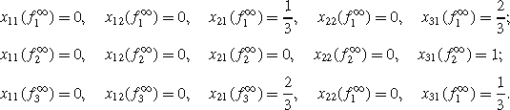Since $$x(f_{1}^{\infty}) = \frac{1}{2}x(f_{2}^{\infty}) +\frac{1}{2}x(f_{3}^{\infty})$$, $$x(f_{1}^{\infty})$$ is not an extreme point of XY.

In order to solve the CMDP (14) we consider the linear program

$$\max\left\{\sum_{i,a} r_i(a) x_i(a)\left\vert \begin{array}{l@{\quad}l}\sum_{i,a} \{\delta_{ij} - p_{ij}(a)\}x_i(a) = 0, &j \in S\\ [0.1cm]\sum_a x_j(a) + \sum_{i,a} \{\delta_{ij} - p_{ij}(a)\}y_i(a) = \beta _j, & j \in S\\ [0.1cm]\sum_{i,a} c^k_i(a) x_i(a) \leq b_k,&1 \leq k \leq m\\ [0.1cm]x_i(a),y_i(a) \geq0, &(i,a) \in S \times A\end{array}\right\}. \right.$$
(20)

The next theorem shows how an optimal policy for the CMDP (14) can be computed. This policy may lie outside the set of stationary policies.

### Theorem 9

1. (1)

Problem (14) is feasible if and only if problem (20) is feasible.

2. (2)

The optima of (14) and (20) are equal.

3. (3)

If R is optimal for problem (14), then x(R) is optimal for (20).

4. (4)

Let (x,y) be an optimal solution of problem (20) and let $$x = \sum_{k=1}^{n} p_{k}x(f_{k})$$, where p k ≥0 and $$\sum_{k=1}^{n} p_{k} = 1$$ and $$C(D) =\{f^{\infty}_{1},f^{\infty}_{2},\dots,f^{\infty}_{n}\}$$. Let RC(M) such that j β j ⋅ℙ R {X t =i,Y t =aX 1}=∑ j β j ⋅∑ k p k $$\mathbb{P}_{f^{\infty}_{k}}\{X_{t} = i, Y_{t} = a\mid X_{1}\}=\beta_{j}\}$$ for all (i,a)∈S×A and all t∈ℕ. Then, R is an optimal solution of problem (14).

To compute an optimal policy from an optimal solution (x,y) of the linear program (20), we first have to express x as $$x =\sum_{k=1}^{n} p_{k}x(f_{k}^{\infty})$$, where p k ≥0 and $$\sum_{k=1}^{n}p_{k} = 1$$. Next, we have to determine the policy R=(π 1,π 2,…)∈C(M) such that R satisfies $$\sum_{j} \beta_{j}\times\mathbb{P}_{R} \{X_{t} = i,Y_{t} = a|X_{1}\} = \sum_{j} \beta_{j} \cdot \sum_{k}p_{k} \cdot\mathbb{P}_{f^{\infty}_{k}}\{X_{t} = i,Y_{t} = a|X_{1}\} = \beta_{j}\}$$ for all (i,a)∈S×A and all t∈ℕ. The decision rules π t,t∈ℕ, can be determined by

$$\pi^t_{ia} = \left\{\begin{array}{l@{\quad}l}\frac{\sum_j \beta_j \cdot\sum_k p_k \{P^{t-1}(f_k)\}_{ji} \cdot \delta_{af_k(i)}}{\sum_j \beta_j \cdot\sum_k p_k \{P^{t-1}(f_k)\}_{ji}}& \mbox{if}\ \sum_j \beta_j \cdot\sum_k p_k \{P^{t-1}(f_k)\}_{ji}\not= 0\\ [0.15cm]\mbox{arbitrary} & \mbox{if}\ \sum_j \beta_j \cdot\sum_k p_k \{P^{t-1}(f_k)\}_{ji} = 0.\end{array}\right.$$

Hence, the following algorithm constructs a policy RC(M)∩C 1 which is optimal for CMDP problem (14).

### Algorithm 2

1. 1.

Determine an optimal solution (x ,y ) of linear program (20) (if (20) is infeasible, then problem (14) is also infeasible).

2. 2.
1. (a)

Let $$C(D) = \{f^{\infty}_{1},f^{\infty}_{2},\ldots,f^{\infty}_{n}\}$$ and compute P (f k ) for k=1,2,…,n.

2. (b)

Take

$$x^k_{ia} = \left\{\begin{array}{l@{\quad}l}\sum_j \beta_j \cdot\{P^*(f_k)\}_{ji} & a = f_k(i)\\ [0.06cm]0 & a \not= f_k(i)\end{array}, \right.\quad i \in S,\ k = 1,2,\dots,n.$$
3. 3.

Determine p k , k=1,2,…,n as feasible solution of the linear system

$$\left\{\begin{array}{l@{\quad}l}\sum_{k=1}^n p_k x^k_{ia} = x^*_{ia}, & a \in A(i),i \in S\\ [0.06cm]\sum_{k=1}^n p_k = 1 \\ [0.06cm]p_k \geq0 & k = 1,2,\dots,n\end{array}\right.$$
4. 4.

R=(π 1,π 2,…), defined by

$$\pi^t_{ia} = \left\{\begin{array}{l@{\quad}l}\frac{\sum_j \beta_j \cdot\sum_k p_k \{P^{t-1}(f_k)\}_{ji} \cdot \delta_{af_k(i)}}{\sum_j \beta_j \cdot\sum_k p_k \{P^{t-1}(f_k)\}_{ji}} & \mbox{if}\ \sum_j \beta_j \cdot\sum_k p_k \{P^{t-1}(f_k)\}_{ji} \not= 0\\ [0.06cm]\mbox{arbitrary} & \mbox{if}\ \sum_j \beta_j \cdot\sum_k p_k \{P^{t-1}(f_k)\}_{ji} = 0\end{array}\right.$$

is an optimal policy for problem (14).

In the next example Algorithm 2 is applied on a CMDP.

### Example 7

Let S={1,2,3}; A(1)={1,2}, A(2)={1}, A(3)={1,2}; p 12(1)=p 13(2)=p 22(1)=p 33(1)=p 32(2)=1 (other transitions are 0); r 1(1)=0, r 1(2)=0, r 2(1)=1, r 3(1)=r 3(2)=0; $$\beta_{1} = \frac{1}{4}$$, $$\beta_{2} = \frac{3}{16}$$, $$\beta_{3} =\frac{9}{16}$$. As constraints we have bounds for the value $$x_{21}(R):\frac{1}{4} \leq x_{21}(R) \leq\frac{1}{2}$$. If we apply Algorithm 2 we obtain the following.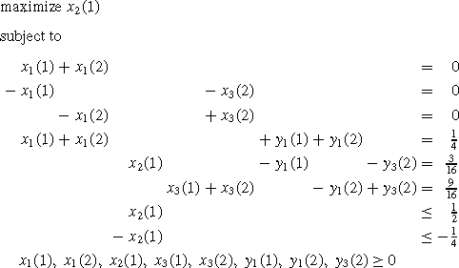with optimal solution: $$x^{*}_{1}(1) = 0$$, $$x^{*}_{1}(2) = 0$$, $$x^{*}_{2}(1) =\frac{1}{2}$$, $$x^{*}_{3}(1) = \frac{1}{2}$$, $$x^{*}_{3}(2) = 0$$; $$y^{*}_{1}(1) = 0$$, $$y^{*}_{1}(2) = \frac{1}{4}$$, $$y^{*}_{3}(2) =\frac{5}{16}$$.

There are four deterministic policies: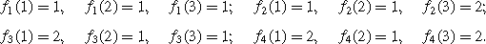The corresponding vectors x 1, x 2, x 3, x 4 are: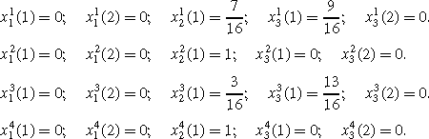For the numbers p 1,p 2,p 3,p 4≥0 such that p 1 x 1+p 2 x 2+p 3 x 3+p 4 x 4=x and $$\sum_{k=1}^{4} p_{k} = 1$$, we obtain: $$p_{1} = \frac{8}{9}$$, $$p_{2} = \frac{1}{9}$$, p 3=0, p 4=0.

Sincewe obtain R=(π 1,π 2,…) with $$\pi^{t}_{11} = 1$$, t∈ℕ; $$\pi^{t}_{21} = 1$$, t∈ℕ;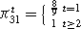;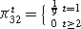.

### Remark

Algorithm 2 is unattractive for practical problems. The number of calculations is prohibitive. Moreover, the use of Markov policies is inefficient in practice. Therefore, we also analyze the problem of finding an optimal stationary policy, if one exists.

For any feasible solution (x,y) of (20) we define a stationary policy π (x,y) in a slightly different way as by (9). The difference is caused by the fact that for constrained MDPs β j can be equal to zero in one or more states j, while in unconstrained MDPs we take β j >0 for all states j.

$$\pi_{ia}(x,y) = \left\{\begin{array}{l@{\quad}l}x_i(a)/x_i & \mbox{if}\ \sum_a x_i(a) > 0 \\[0.1cm]y_i(a)/y_i & \mbox{if}\ \sum_a x_i(a) = 0\ \mbox{and}\ \sum_ay_i(a) > 0 \\[0.1cm]\mbox{arbitrary} &\mbox{if}\ \sum_a x_i(a) = 0\ \mbox{and}\ \sum _a y_i(a) = 0 .\end{array}\right.$$
(21)

In Kallenberg (1983) the following lemmata can be found.

### Lemma 3

If (x ,y ) is an optimal solution of problem (20) and the Markov chain P(π(x ,y )) has one ergodic set plus a (perhaps empty) set of transient states, then π (x ,y ) is an optimal policy for problem (14).

### Lemma 4

If (x ,y ) is an optimal solution of problem (20) and x satisfies $$x^{*}_{i}(a) = \pi_{ia}(x^{*},y^{*}) \cdot\{\beta^{T} P^{*}(\pi(x^{*},y^{*}) )\}_{i}$$ for all (i,a)∈S×A, then π (x ,y ) is an optimal policy for problem (14).

### Lemma 5

If (x ,y ) is an optimal solution of problem (20) and furthermore $$x^{*}_{i}(a)/x^{*}_{i} = y^{*}_{i}(a)/y^{*}_{i}$$ for all pairs (i,a) with iS +, aA(i), where $$x^{*}_{i} = \sum_{a} x^{*}_{ia}$$, $$y^{*}_{i}= \sum_{a} y^{*}_{ia}$$ and $$S_{+} = \{i\mid x^{*}_{i} > 0, y^{*}_{i} > 0\}$$, then the stationary policy π (x ,y ) is an optimal policy for problem (14).

The next example shows that for an optimal solution (x ,y ) of (20), the policy π (x ,y ) is not an optimal solution of (14), even in the case that (14) has a stationary optimal policy.

### Example 7

(continued)

Consider the MDP model of Example 7, but with as constraint $$x_{21}(R) \leq\frac{1}{4}$$. The linear program (20) for this constrained problem is: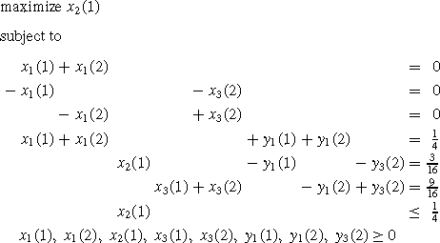with optimal solution $$x^{*}_{1}(1) = 0$$, $$x^{*}_{1}(2) = 0$$, $$x^{*}_{2}(1) =\frac{1}{4}$$, $$x^{*}_{3}(1) = \frac{3}{4}$$, $$x^{*}_{3}(2) = 0$$; $$y^{*}_{1}(1) =0$$, $$y^{*}_{1}(2) = \frac{1}{4}$$, $$y^{*}_{3}(2) = \frac{1}{16}$$ and with optimum value $$\frac{1}{4}$$. The corresponding stationary policy π (x ,y ) gives π 12=π 21=π 31=1, so this policy is in fact deterministic. This policy is not optimal, because $$\phi (\pi^{\infty}(x^{*},y^{*}) ) = \frac{3}{16} < \frac{1}{4}$$, the optimum of the linear program. Consider the stationary policy π with $$\pi_{11} = \frac{1}{4}$$, $$\pi_{12} =\frac{3}{4}$$, π 21=π 31=1. For this policy we obtain $$x_{12}(\pi^{\infty}) = \frac{1}{4}$$ and $$\phi(\pi^{\infty}) =\frac{1}{4}$$, the optimum value of the linear program. So, this policy is feasible and optimal.

If the conditions of Lemma 5 are not satisfied, we can try to find for the same x another y , say $$\overline{y}$$, such that $$(x^{*},\overline{y})$$ is feasible for (20), and consequently also optimal, and satisfies the conditions of Lemma 5. To achieve this, we need $$\overline{y}_{i}(a)/\overline{y}_{i} = \pi_{ia}$$, aA(i), $$i \in\{j\mid x^{*}_{j} > 0, \overline{y}_{j} > 0\}$$, which is equivalent to $$\overline{y}_{i}(a) = \overline{y}_{i} \cdot\pi_{ia}$$, aA(i), $$i \in\{j\mid x^{*}_{j} > 0\}$$. Hence, $$\overline{y}$$ has to satisfy the following linear system in the y-variables (x is fixed)

$$\left\{\begin{array}{l}\sum_{i \notin S_*} \sum_a \{\delta_{ij} - p_{ij}(a)\}\overline{y}_i(a)+ \sum_{i \in S_*} \{\delta_{ij} - p_{ij}(\pi)\}\overline{y}_i =\beta_j - x^*_j,\quad j \in S\\ [0.15cm]\overline{y}_i(a) \geq0, i \notin S_*,a \in A(i); \overline{y}_i \geq0, i \in S_*,\quad \mbox{with}\ S_* = \{j |\sum_a x^*_j(a) > 0\}.\end{array}\right.$$
(22)
• Example 7 (continued)

• The optimal solution (x ,y ) with $$x^{*}_{1}(1) = 0$$, $$x^{*}_{1}(2) = 0$$, $$x^{*}_{2}(1) = \frac{1}{4}$$, $$x^{*}_{3}(1) = \frac{3}{4}$$, $$x^{*}_{3}(2) = 0$$; $$y^{*}_{1}(1) = 0$$, $$y^{*}_{1}(2) =\frac{1}{4}$$, $$y^{*}_{3}(2) = \frac{1}{16}$$ does not satisfy $$x^{*}_{i}(a)/x^{*}_{i} = y^{*}_{i}(a)/y^{*}_{i}$$ for all aA(i), iS +, because S +={3} and $$x^{*}_{3}(2)/x^{*}_{3} = 0$$ and $$y^{*}_{3}(2)/y^{*}_{3} = 1$$. The system (22) becomes $$\overline{y}_{1}(1) + \overline{y}_{1}(2) = \frac{4}{16}$$; $$-\overline{y}_{1}(1) = -\frac{1}{16}$$; $$-\overline{y}_{1}(2) = -\frac{3}{16}$$; $$\overline{y}_{1}(1), \overline{y}_{1}(2) \geq0$$. This system has the solution $$\overline{y}_{1}(1) = \frac{1}{16}$$, $$\overline{y}_{1}(2) = \frac{3}{16}$$. The stationary policy π with $$\pi_{11} = \frac{1}{4}$$, $$\pi_{12} = \frac{3}{4}$$, π 21=π 31=1 is optimal for problem (14).

### Remark

If the x-part of problem (20) is unique and (22) is infeasible, then problem (14) has no optimal stationary policy. If the x-part of problem (20) is not unique and (22) is infeasible, then it is still possible that there exists an optimal stationary policy. In that case we can compute every extreme optimal solution of the linear program (20), and for each of these extreme optimal solutions we can perform the above analysis in order to search for an optimal stationary policy. We show an example of this approach.

### Example 8

Take the MDP with S={1,2,3}; A(1)={1,2}, A(2)={1,2}, A(3)={1}; p 12(1)=p 13(2)=p 22(1)=p 21(2)=p 33(1)=1 (other transitions are 0). r 1(1)=r 1(2)=0, r 2(1)=1, r 2(2)=0, r 3(1)=1. Let $$\beta_{1} = \beta_{2} = \beta_{3} = \frac{1}{3}$$. Add as only constraint $$x_{21}(R) \geq\frac{1}{9}$$. The formulation of the linear program (20) becomes: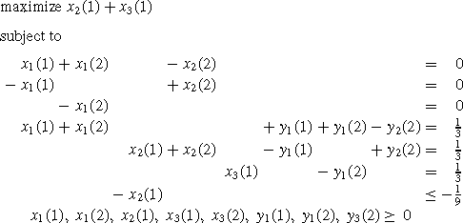with extreme optimal solution $$x^{*}_{1}(1) = 0$$, $$x^{*}_{1}(2) = 0$$, $$x^{*}_{2}(1)= \frac{1}{9}$$, $$x^{*}_{2}(2) = 0$$, $$x^{*}_{3}(1) = \frac{8}{9}$$; $$y^{*}_{1}(1) =0$$, $$y^{*}_{1}(2) = \frac{5}{9}$$, $$y^{*}_{2}(2) = \frac{2}{9}$$ and with optimum value 1. The x-part of this problem is not unique. It can easily be verified that $$\hat{x}_{1}(1) = 0$$, $$\hat{x}_{1}(2) = 0$$, $$\hat{x}_{2}(1) =\frac{2}{3}$$, $$\hat{x}_{2}(2) = 0$$, $$\hat{x}_{3}(1) =\frac{1}{3}$$; $$\hat{y}_{1}(1) = \frac{1}{3}$$, $$\hat{y}_{1}(2) = 0$$, $$\hat{y}_{2}(2) = 0$$ is also an extreme optimal solution. For the first extreme optimal solution (x ,y ) system (22) becomes

$$\overline{y}_1(1) + \overline{y}_1(2) = \frac{1}{3};\quad -\overline{y}_1(1) = \frac{2}{9}; \quad\overline{y}_1(2) = -\frac {5}{9};\quad \overline{y}_1(1), \overline{y}_1(2) \geq0.$$

This system is obviously infeasible.

For the second extreme optimal solution $$(\hat{x},\hat{y})$$ we can apply Lemma 5, which gives that the deterministic policy $$f_{*}^{\infty}$$ with f (1)=f (2)=f (3)=1 is an optimal solution.

### Remarks

1. 1.

These problems have always a stationary optimal policy. The analysis for this kind of problems is much easier than for MDPs with the average reward as optimality criterion (see Kallenberg 2010).

2. 2.

Multiple objectives

Some problems may have several kinds of rewards or costs, which cannot be optimized simultaneously. Assume that we want to maximize some utility for an m-tuple of immediate rewards, say utilities u k(R) and immediate rewards $$r_{i}^{k}(a)$$, (i,a)∈S×A, for k=1,2,…,m. For each k one can find an optimal policy R k , i.e., $$u_{i}^{k}(R_{k})\geq u_{i}^{k}(R)$$, iS, for all policies R. However, in general, $$R_{k} \not= R_{l}$$ if $$k \not=l$$, and there does not exist one policy which is optimal for all m rewards simultaneously for all starting states. Therefore, we consider the utility function with respect a given initial distribution β. Given this initial distribution β and a policy R, we denote the utilities by u k(β,R). The goal in multi-objective optimization is to find an β-efficient solution, i.e., a policyR such that there exists no other policy R satisfying u k(β,R)≥u k(β,R ) for all k and u k(β,R)>u k(β,R ) for at least one k. These problems can be solved, for both discounted rewards and average rewards, by CMDPs (for more details, see Kallenberg 2010).

## 5 Applications

### 5.1 Optimal stopping problems

In Chap. 8 of Derman’s book (Derman 1970) optimal stopping of a Markov chain is discussed. Derman considers the following model. Let {X t ,t=1,2,…} be a finite Markov chain with state space S and stationary transition probabilities p ij . Let us suppose there exists an absorbing state 0, i.e., p 00=1, such that ℙ{X t =0 for some t≥1∣X 1=i}=1 for every iS. Let r i , iS, denote nonnegative values.

When the chain is absorbed at state 0, we can think of the process as having been stopped at that point in time and we receive the value r 0. However, we can also think of stopping the process at any point in time prior to absorption and receiving the value r i if i is the state of the chain when the process is stopped. If our aim is to receive the highest possible value and if r 0<max iS r i , then clearly we would not necessarily wait for absorption before stopping the process.

By a stopping time τ, we mean a rule that prescribes the time to stop the process. Optimal stopping of a Markov chain is the problem to determine the stopping time τ such that $$\mathbb{E}\{r_{X_{\tau}}\mid X_{1} = i\}$$ is maximized for all iS. Let $$M_{i} = \max_{\tau}\mathbb{E}\{r_{X_{\tau}}\mid X_{1} = i\}$$, iS. Derman has shown the following result.

### Theorem 10

If v is an optimal solution of the linear program

$$\min\left\{\sum_j v_j \left\vert \begin{array}{l@{\quad}l}v_i \geq r_i, & i \in S\\ [0.1cm]v_i \geq\sum_j p_{ij}v_j, & i \in S\end{array}\right\}, \right.$$
(23)

then $$M_{i} = v^{*}_{i}$$, iS.

In Kallenberg (1983) this approach is generalized in the following way:

• the assumption r i ≥0, iS, is omitted;

• if we continue in state i, a cost c i is incurred for all iS;

• we can determine not only M i , iS, but also the states S 0 in which it is optimal to stop.

The results are based on properties for convergent MDPs with as optimality criterion the total expected reward over an infinite horizon. The following theorem shows the result.

### Theorem 11

Let v and (x ,y ) be optimal solutions of the following dual pair of linear programs

$$\min\left\{\sum_j v_j \left\vert \begin{array}{l@{\quad}l}v_i \geq r_i, & i \in S \\ [0.1cm]v_i \geq-c_i + \sum_j p_{ij}v_j, & i \in S\end{array}\right\} \right.$$
(24)

and

$$\max\left\{\sum_i r_ix_i - \sum_i c_iy_i \left\vert \begin{array}{l@{\quad}l}x_j + y_j - \sum_i p_{ij}y_i = 1,& j \in S \\x_i, y_i \geq0, & i \in S\end{array}\right\}.\right.$$
(25)

Then, $$M_{i} = v^{*}_{i}$$, iS and $$S_{0} = \{i \in S\mid x^{*}_{i} > 0\}$$.

Furthermore, we have the following result for monotone optimal stopping problems, i.e., problems that satisfy p ij =0 for all iS 1, jS 1, where S 1={iSr i ≥−c i +∑ j p ij r j }. So, S 1 is the set of states in which immediate stopping is not worse than continuing for one period and than choose to stop. The set S 1 follows directly from the data of the model.

### Theorem 12

In a monotone optimal stopping problem a one-step look ahead policy, i.e., a policy that stops in the states of S 1 and continues outside S 1, is an optimal policy.

### 5.2 Replacement problems

#### 5.2.1 General replacement problem

In a general replacement model we have state space S={0,1,…,N}, where state 0 corresponds to a new item, and action sets A(0)={1} and A(i)={0,1}, $$i \not= 0$$, where action 0 means replacing the ‘old’ item by a new item. We consider in this model costs instead of rewards. Let c be the cost of a new item.

Furthermore, assume that an item of state i has trade-in-value s i and maintenance costs c i . If in state i action 0 is chosen, then c i (0)=cs i +c 0 and p ij (0)=p 0j , jS; for action 1, we have c i (1)=c i and p ij (1)=p ij , jS. In contrast with other replacement models, where the state is determined by the age of the item, we allow that the state of the item may change to any other state.

In this case the optimal replacement policy is in general not a control-limit rule. As optimality criterion we consider the discounted reward. For this model the primal linear program is:

$$\min\left\{\sum_{j=0}^N \beta_{j}v_{j} \left\vert \begin{array}{l@{\quad}l}\sum_{j=0}^N (\delta_{ij} - \alpha p_{0j})v_j \geq-c + s_i -c_0, &1 \leq i \leq N\\[0.1cm]\sum_{j=0}^N (\delta_{ij} - \alpha p_{ij})v_j \geq-c_i, & 0 \leq i\leq N\end{array}\right.\right\},$$
(26)

where β j >0, jS. Because there is only one action in state 0, namely action 1, we have $$v^{\alpha}_{0} = -c_{0} + \alpha \sum^{N}_{j=0} p_{0j}v^{\alpha}_{j}$$.

Hence, instead of $$v_{i} - \alpha\sum^{N}_{j=0} p_{0j}v_{j} =\sum^{N}_{j=0}$$ (δ ij αp 0j )v j ≥−c+s i c 0, we can write v i v 0≥−c+s i , obtaining the equivalent linear program

$$\min\left\{\sum_{j=0}^N \beta_{j}v_{j} \left\vert \begin{array}{l@{\quad}l}v_i - v_0 \geq r_i, & 1 \leq i \leq N\\[0.1cm]\sum_{j=0}^N (\delta_{ij} - \alpha p_{ij})v_{j} \geq-c_i,& 0 \leq i\leq N\end{array}\right.\right\},$$
(27)

where r i =−c+s i , iS. The dual linear program of (27) is:

$$\max\left\{\sum_{i=1}^N r_i x_i - \sum_{i=0}^N c_i y_i \left\vert \begin{array}{l@{\quad}l}-\sum_{i=1}^N x_i + \sum_{i=0}^N (\delta_{i0} - \alpha p_{i0})y_i =\beta_0 \\[0.1cm]x_j + \sum_{i=0}^N (\delta_{ij} - \alpha p_{ij})y_i = \beta_j, & 1\leq j \leq N\\[0.1cm]x_i \geq0, & 1 \leq i \leq N\\[0.1cm]y_i \geq0, & 0 \leq i \leq N\end{array}\right.\right\}.$$
(28)

For this linear program the following result can be shown. For the proof we refer to Kallenberg (2010).

### Theorem 13

There is a one-to-one correspondence between the extreme solutions of (28) and the set of deterministic policies.

Consider the simplex method to solve (28) and start with the basic solution that corresponds to the policy which chooses action 1 (no replacement) in all states. Hence, in the first simplex tableau y j , 0≤jN, are the basic variables and x i , 1≤iN, the nonbasic variables. Take the usual version of the simplex method in which the column with the most negative cost is chosen as pivot column. It turns out, see Theorem 14, that this choice gives the optimal action for that state, i.e., in that state action 0, the replacement action, is optimal. Hence, after interchanging x i and y i , the column of y i can be deleted. Consequently, we obtain the following greedy simplex algorithm.

### Algorithm 3

(Greedy simplex algorithm)

1. 1.

2. 2.

If the reduced costs are nonnegative: the corresponding policy is optimal (STOP).

Otherwise:

1. (a)

Choose the column with the most negative reduced cost as pivot column.

2. (b)

Execute the usual simplex transformation and delete the pivot column.

3. 3.

If all columns are removed: replacement in all states is the optimal policy (STOP).

### Theorem 14

The greedy simplex algorithm is correct and has complexity $$\mathcal{O}(N^{3})$$.

### Remark 1

For the proof of Theorem 14 we also refer to Kallenberg (2010). The linear programming approach, as discussed in this section, is related to a paper by Gal (1984), in which the method of policy iteration was considered.

### Remark 2

An optimal stopping problem may be considered as a special case of a replacement problem with as optimality criterion the total expected reward, i.e., α=1. In an optimal stopping problem there are two actions in each state. The first action is the stopping action and the second action corresponds to continue. If the stopping action is chosen in state i, then a final reward r i is earned and the process terminates. If the second action is chosen, then a cost c i is incurred and the transition probability of being in state j at the next decision time point is p ij , jS. This optimal stopping problem is a special case of the replacement problem with p 0j =0 for all jS, c i (0)=−r i and c i (1)=c i for all iS. Hence, also for the optimal stopping problem, the linear programming approach of this section can be used and the complexity is also $$\mathcal{O}(N^{3})$$.

### Remark 3

With a similar approach, the average reward criterion for an irreducible general replacement problem can be treated.

#### 5.2.2 Replacement problem with increasing deterioration

Consider a replacement model with state space S={0,1,…,N+1}. An item is in state 0 if and only if it is new; an item is in state N+1 if and only if it is inoperative. In states 1,2,…,N there are two actions: action 0 is to replace the item by a new one and action 1 is not to replace the item. In the states 0 and N+1 only one action is possible (no replacement and replacement by a new item, respectively) and call this action 1 and 0, respectively. The transition probabilities are:

$$p_{ij}(0) = \left\{\begin{array}{l@{\quad}l}0, & 1 \leq i \leq N + 1,\ j \not= 0 \\[0.1cm]1, & 1 \leq i \leq N +1,\ j = 0\end{array}\right.;\quad p_{ij}(1) = p_{ij},\ 0 \leq i \leq N,\ 1 \leq j \leq N+1.$$

We assume two types of cost, the cost c 0≥0 to replace an operative item by a new one and the cost c 0+c 1, where c 1≥0, to replace an inoperative item by a new one. Thus, c 1 is the additional cost incurred if the item becomes inoperative before being replaced. Hence, the costs c are:

$$c_i(0) = c_0,\quad1 \leq i \leq N;\quad c_{N+1}(0) = c_0 + c_1;\quad c_i(1) = 0,\quad0 \leq i \leq N.$$

We state the following assumptions, which turn out to be equivalent (see Lemma 6).

### Assumption 1

The transition probabilities are such that for every nondecreasing function x j , jS, the function $$F(i) = \sum_{j=0}^{N+1}p_{ij}x_{j}$$ is nondecreasing in i.

### Assumption 2

The transition probabilities are such that for every kS, the function $$G_{k}(i) = \sum_{j=k}^{N+1} p_{ij}$$ is nondecreasing in i.

### Lemma 6

The Assumptions 1 and 2 are equivalent.

The significance of Lemma 6 is that Assumption 1 can be verified by the verification of Assumption 2, which can be verified only using the data of the model. Assumption 2 means that this replacement model has increasing deterioration.

We first consider the criterion of discounted costs. For this criterion the following result can be shown, which is based on the property that the value vector $$v^{\alpha}_{i}$$, 0≤iN+1, is nondecreasing in the states i.

### Theorem 15

If Assumption 1 (or 2) holds and if the state i is such that $$i_{*} = \max\{i\mid\alpha \sum_{j}p_{ij}v_{j}^{\alpha}\leq c_{0} + \alpha\sum_{j} p_{0j}v_{j}^{\alpha}\}$$. Then, the control-limit policy $$f^{\infty}_{*}$$ which replaces in the states i>i is a discounted optimal policy.

Theorem 15 implies that the next algorithm computes an optimal control-limit policy for this model. Similar to Algorithm 3 it can be shown that the complexity of Algorithm 4 is $$\mathcal {O}(N^{3})$$.

### Algorithm 4

(Computation of an optimal control-limit policy)

1. 1.
1. (a)

Start with the basic solution corresponding to the nonreplacing actions in the states i=1,2,…,N and to the only action in the states 0 and N+1.

2. (b)

Let k=N (the number of nonbasic variables corresponding to the replacing actions in the states i=1,2,…,N).

2. 2.

If the reduced costs are nonnegative: the corresponding policy is optimal (STOP).

Otherwise:

1. (a)

Choose the column corresponding to state k as pivot column.

2. (b)

Execute the usual simplex transformation.

3. (c)

Delete the pivot column.

3. 3.

If all columns are removed: replacement in all states is the optimal policy (STOP).

Next, we consider the criterion of average cost. By Theorem 15, for each α∈(0,1) there exists a control-limit policy $$f^{\infty}_{\alpha}$$ that is α-discounted optimal. Let {α k ,k=1,2,…} be any sequence of discount factors such that lim k→∞ α k =1.

Since there are only a finite number of different control-limit policies, there is a subsequence with one of these policies. Therefore, we may assume that $$f^{\infty}_{\alpha_{k}} = f^{\infty}_{0}$$ for all k. Let f be any policy in C(D). Since $$f^{\infty}_{0} =f^{\infty}_{\alpha_{k}}$$ is optimal for all k, we have

$$(1 - \alpha_k)v^{\alpha_k}(f^\infty) \geq(1 - \alpha_k)v^{\alpha _k}(f^\infty_0)\quad\mbox{for}\ k = 1,2,\dots.$$

Letting k→∞, we obtain for every f C(D),

$$\phi(f^\infty) = \lim_{k \rightarrow\infty} (1 - \alpha _k)v^{\alpha_k}(f^\infty)\geq\lim_{k \rightarrow\infty} (1 - \alpha_k)v^{\alpha _k}(f^\infty_0)= \phi(f^\infty_0).$$

Therefore, the following result holds.

### Theorem 16

If Assumption 1 (or 2) holds, then there exists a control-limit policy $$f^{\infty}_{*}$$ such that $$\phi(f^{\infty}_{*}) \leq\phi(f^{\infty})$$ for all policies f C(D).

### Remark

The results of this section, with the exception of Algorithm 4, have been developed by Derman (1963).

This model is slightly different from the previous one, replacement with increasing deterioration. Let the state space S={0,1,…,N+1}, where state 0 corresponds to a new item and state N+1 to failure. The states i, 0≤iN, may be interpreted as the age of the item. The system has in state i (0≤iN) a failure probability p i during the next period. When failure occurs in state i, which is modeled as being transferred to state N+1, there is an additional cost f i . In state N+1 the item has to be replaced by a new one. In the states 1≤iN there are two actions. Action 0 replaces the item immediately by a new one, so it has the same transitions as state 0; the replacement cost is c. By action 1 the system moves, when there is no failure, from state i to the next state i+1: the system skips to the right, i.e., the age of the item increases. Furthermore, in state i there are maintenance cost c i .

The action sets, the cost of a new item, the maintenance costs and the transition probabilities are as follows.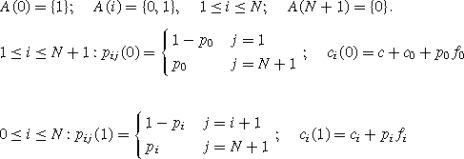We impose the following assumptions:

1. (A1)

c≥0; c i ≥0, f i ≥0, 0≤iN.

2. (A2)

p 0p 1≤⋯≤p N , i.e., older items have greater failure probability.

3. (A3)

c 0+p 0 f 0c 1+p 1 f 1≤⋯≤c N +p N f N , i.e., the expected maintenance and failure costs grow with the age of the item.

Take any kS. Since

$$\sum_{j=k}^{N+1} p_{ij}(1) = \left\{\begin{array}{l@{\quad}l}p_i & i \leq k-2\\[0.1cm]1 & i \geq k-1\\\end{array}\right.,$$

this summation is, by assumption A2, nondeceasing in i. Hence, Assumption 2 and consequently also Assumption 1 of the previous section, is satisfied. This enables us to treat this model in a similar way as the model with increasing deterioration. In this way we can derive the following result.

### Theorem 17

Let the assumptions (A1), (A2) and (A3) hold, and let $$i_{*} =\max\{i\mid c_{i} + p_{i}f_{i} + \alpha\sum_{j} p_{ij}(1)v_{j}^{\alpha}\leq c+c_{0} +p_{0}f_{0} + \alpha\sum_{j} p_{0j}(1)v_{j}^{\alpha}\}$$. Then, the control-limit policy $$f^{\infty}_{*}$$ which replaces in the states i>i is an optimal policy.

### Remarks

1. 1.

For the proof of Theorem 17 we refer to Kallenberg (1994).

2. 2.

Algorithm 4 is also applicable to this model.

3. 3.

Similarly as in the previous section it can be shown that for the average cost criterion there exists also a control-limit optimal policy.

4. 4.

In Derman (1970, pp. 125–130) a surveillance-maintenance-replacement model is discussed. This model is solved in the following way:

1. (a)

A fractional linear programming formulation is developed from which an optimal policy can be derived.

2. (b)

This fractional linear programming can be transformed into a normal linear program. This transformation is due to Derman and his student Klein (see Derman 1962 and Klein 1962). See Charnes and Cooper (1962) and Wagner and Yuan (1968) for more general treatment of linear fractional programming.

#### 5.2.4 Separable replacement problem

Suppose that the MDP has the following structure: S={0,1,2,…,N}; A(i)={1,2,…,M}, iS; p ij (a)=p j (a), i,jS, aA(i), i.e., the transitions are state independent; r i (a)=s i +t(a), iS, aA(i), i.e., the rewards are separable.

As example, consider the problem of periodically replacing a car. The age of a car can be 0,1,…,N. When a car is replaced, it can be replaced not only by a new one (state 0), but also by a car in an arbitrary state a, 1≤aN. Let s i be the trade-in-value of a car of state i, t(a) the costs of a car of state a. Then, r i (a)=s i t(a) and p ij (a)=p j (a), where p j (a) is the probability that a car of state a is in state j at the next decision time point.

The next theorems show that a one-step look ahead policy is optimal both for discounted as for undiscounted rewards.

### Theorem 18

The policy $$f^{\infty}_{1}$$, defined by f 1(i)=a 1 for all i, where a 1 is such thatt(a 1)+α j p j (a 1)s j =max1≤aM {−t(a)+α j p j (a)s j }, is an α-discounted optimal policy.

### Theorem 19

The policy $$f^{\infty}_{2}$$, defined by f 2(i)=a 2 for all i, where a 2 is such thatt(a 2)+∑ j p j (a 2)s j =max1≤aM {−t(a)+∑ j p j (a)s j }, is an average optimal policy.

### 5.3 Multi-armed bandit problems

#### 5.3.1 Introduction

The multi-armed bandit problem is a model for dynamic allocation of a resource to one of n independent alternative projects. Any project may be in one of a finite number of states, say project j in the set S j , j=1,2,…,n. Hence, the state space S is the Cartesian product S=S 1×S 2×⋯×S n . Each state i=(i 1,i 2,…,i n ) has the same action set A={1,2,…,n}, where action k means that project k is chosen, k=1,2,…,n. So, at each stage one can be working on exactly one of the projects.

When project k is chosen in state i—the chosen project is called the active project—the immediate reward and the transition probabilities only depend on the active project, whereas the states of the remaining projects are frozen. Let $$r_{i_{k}}$$ and $$p_{i_{k}j}$$, jS k denote these quantities when action k is chosen. The total discounted reward criterion is chosen.

It was shown by Gittins and Jones (1974, 1979) that an optimal policy is the policy that selects project k in state i=(i 1,i 2,…,i n ), where k satisfies

$$G_k(i_k) = \max_{1 \leq j \leq n}~G_j(i_j)$$

for certain numbers G j (i j ), i j S j , 1≤jn. Such a policy is called an index policy. Surprisingly, the number G j (i j ) only depends on project j and not on the other projects. These indices are called the Gittins indices.

As a consequence, the multi-armed bandit problem can be solved by solving a sequence of n one-armed bandit problems. This is a decomposition result by which the dimensionality of the problem is reduced considerably. Algorithms with complexity $$\mathcal{O}(\sum_{j=1}^{n} n_{j}^{3})$$, where n j =|S j |, 1≤jn, do exist for the computation of all indices.

#### 5.3.2 A single project with a terminal reward

Consider the one-armed bandit problem with stopping option, i.e., in each state there are two options: action 1 is the stopping option and then one earns a terminal reward M and by action 2 the process continues with in state i an immediate reward r i and transition probabilities p ij . Let v α(M) be the value vector of this optimal stopping problem. Then, v α(M) is the unique solution of the optimality equation

$$v_i^\alpha(M) = \max\biggl\{M, r_i + \alpha\sum_j p_{ij}v_j^\alpha (M)\biggr\},\quad i \in S,$$
(29)

and of the linear program

$$\min\left\{\sum_j v_j \left\vert \begin{array}{l@{\quad}l}\sum_j \{\delta_{ij} - \alpha p_{ij}\}v_j \geq r_i, & i \in S \\ [0.1cm]v_i \geq M, & i \in S \\ [0.1cm]\end{array}\right. \right\}.$$
(30)

Furthermore, we have the following results.

### Theorem 20

Let (x,y) be an extreme optimal solution of the dual program of (30), i.e.,

$$\max\left\{\sum_j r_i x_i + M \cdot\sum_j y_i \left\vert \begin{array}{l@{\quad}l}\sum_i \{\delta_{ij} - \alpha p_{ij}\}x_i + y_j = 1, & i \in S \\ [0.1cm]x_i, y_i \geq0, & i \in S \\ [0.1cm]\end{array}\right. \right\}.$$
(31)

Then, the policy f such that

$$f(i) = \left\{\begin{array}{l@{\quad}l}2 & \mbox{if}\ x_i > 0 \\[0.1cm]1 & \mbox{if}\ x_i = 0\end{array}\right.$$

is an optimal policy.

### Lemma 7

$$v_{i}^{\alpha}(M) - M$$ is a nonnegative continuous nonincreasing function in M, for all iS.

Define the indices G i , iS, by $$G_{i} = \min\{M\mid v^{\alpha}_{i}(M) =M\}$$. Hence, $$v^{\alpha}_{i}(G_{i}) = G_{i}$$ and, by Lemma 7, $$v^{\alpha}_{i}(M) = M$$ for all MG i . For these indices one can show the following theorem.

### Theorem 21

For any M, the policy f C(D) which chooses the stopping action in state i if and only if MG i is optimal.

For M=G i both actions (stop or continue) are optimal. Hence, an interpretation of the Gittins index G i is that it is the terminal reward under which in state i both actions are optimal. Therefore, this number is also called the indifference value.

#### 5.3.3 Multi-armed bandits

Consider the multi-armed bandit model with an additional option (action 0) in each state. Action 0 is a stopping option and then one earns a terminal reward M. One can show the following result.

### Theorem 22

For any state i=(i 1,i 2,…,i n ) and any terminal reward M, the policy that takes the stopping action if $$M \geq G_{i_{j}}$$ for all j=1,2,…,n and continues with project k if $$G_{i_{k}} = \max_{j}G_{i_{j}} > M$$, is an optimal policy.

The preceding theorem shows that the optimal policy in the multi-project case can be determined by an analysis of the n single-project problems, with the optimal decision in state i=(i 1,i 2,…,i n ) being to operate on that project k having the largest $$G_{i_{k}}$$ if this value is greater than M and to stop otherwise.

Several methods have been proposed for the computation of the Gittins indices. We mention the contributions of Katehakis and Veinott (the restart-in-state method, see Katehakis and Veinott 1987), Varaiya, Walrand and Buyukkoc (the-largest-remaining-index method, see Varaiya et al. 1985), and Chen and Katehakis (the linear programming method, see Chen and Katehakis 1986). In this article we present the parametric linear programming method proposed in Kallenberg (1986). This method has for a project with N states complexity $$\mathcal{O}(N^{3})$$.

#### 5.3.4 The parametric linear programming method

We have already seen that for a single project with terminal reward M the solution can be obtained from a linear programming problem, namely program (31). For M big enough, e.g., for MC=(1−α)⋅max i r i , we know that $$v^{\alpha}_{i}(M) = M$$ for all states i. Furthermore, we have seen that the Gittins index $$G_{i} =\min\{M\mid v^{\alpha}_{i}(M) = M\}$$.

One can solve program (31) as a parametric linear programming problem with parameter M. Starting with M=C one can decrease M and find for each state i the largest M for which it is optimal to keep working on the project, which is in fact $$\min\{M\mid v^{\alpha}_{i}(M)= M\} = G_{i}$$, in the order of decreasing M-values.

One can start with the simplex tableau in which all y-variables are in the basis and in which the x-variables are the nonbasic variables. This tableau is optimal for MC. Decrease M until we meet a basis change, say the basic variable y i will be exchanged with the nonbasic variable x i . Then, we know the M-value which is equal to G i . In this way we continue and repeat the procedure N times, where N is the number of states in the current project. The used pivoting row and column do not influence any further pivoting step, so we can delete these row and column from the simplex tableau.

We can easily determine the computational complexity. Each update of an element in a simplex tableau needs at most two arithmetic operations (multiplication and divisions as well as additions and subtractions). Hence, the total number of arithmetic operations in this method for a project with N states, is at most $$2 \cdot\sum_{k=1}^{N} k^{2} =\frac{1}{3}N(N+1)(2N + 1) = \mathcal{O}(N^{3})$$.

### Remark

The problem of assigning one of several treatments in clinical trials can be formulated as a multi-armed bandit problem. Derman and Katehakis (1987) have used the characterization of the Gittins index as a restart-in-state problem (see Katehakis and Veinott 1987) to calculate efficiently the Gittins values for clinical trials. The characterization of the Gittins index as a restart-in-state problem is related to a general replacement problem as treated by Derman in his book (Derman 1970, pp. 121–125).

### 5.4 Separable problems

#### 5.4.1 Introduction

Separable MDPs have the property that for certain pairs (i,a)∈S×A:

1. (1)

the immediate reward is the sum of two terms, one depends only on the current state and the other depends only on the chosen action: r i (a)=s i +t a .

2. (2)

the transition probabilities depend only on the action and not on the state from which the transition occurs: p ij (a)=p j (a), jS.

Let S 1×A 1 be the subset of S×A for which the pairs (i,a) satisfy (1) and (2). We also assume that the action sets of A 1 are nested: let S 1={1,2,…,m}, then $$A_{1}(1)\supseteq A_{1}(2) \supseteq\cdots\supseteq A_{1}(m) \not= \emptyset$$. Let S 2=S\S 1A 2(i)=A(i)\A 1(i), 1≤im and A 2(i)=A(i), m+1≤iN. We also introduce the notation B(i)=A 1(i)\A 1(i+1), 1≤im−1 and B(m)=A 1(m). Then, $$A_{1}(i) = \bigcup_{j=i}^{m} B(j)$$ and the sets B(j) are disjunct. We allow that S 2, A 2 or B(i), 1≤im−1, are empty sets.

If the system is observed in state iS 1 and the decision maker will choose an action from A 1(i), then, the decision process can be considered as follows. First, a reward s i is earned and the system makes a zero-time transition to an additional state N+i. In this additional state there are two options: either to take an action aB(i) or to take an action aA 1(i)\B(i)=A 1(i+1). In the first case the reward t a is earned and the process moves to state j with probability p j (a), jS; in the second case we are in the same situation as in state N+i, but now in N+i+1, i.e., a zero-time transition is made from state N+i to state N+i+1.

A lot of dynamic decision problems are separable, e.g., the automobile replacement problem which was first considered by Howard (see Howard 1960)

#### 5.4.2 Discounted rewards

The description in the introduction as a problem with zero-time and one-time transitions gives rise to the transformed model with N+m states and to the following linear program for the computation of the value vector v α:

$$\min\left\{\sum_{i=1}^N v_i + \sum_{i=1}^m y_i \left\vert \begin{array}{l@{\quad}l}v_i \geq r_i(a) + \alpha\sum_{j=1}^N p_{ij}(a)v_j, & 1 \leq i \leq N,\ a \in A_2(i) \\ [0.1cm]v_i \geq s_i + y_i, & 1 \leq i \leq m \\ [0.1cm]y_i \geq t_a + \alpha\sum_{j=1}^N p_j(a)v_j, & 1 \leq i \leq m,\ a\in B(i) \\ [0.1cm]y_i \geq y_{i+1}, & 1 \leq i \leq m - 1\end{array}\right. \right\}.$$
(32)

The first set of inequalities corresponds to the non-separable set S×A 2 with one-time transitions; the second set inequalities to the zero-time transitions from the state i to N+i, 1≤im; the third set of inequalities to the set S 1×B with one-time transitions and the last set inequalities corresponds to the zero-time transitions from the state N+i to N+i+1, 1≤im−1.

The dual of program (32), where the dual variables x i (a), λ i , w i (a), ρ i correspond to the four sets of constraints in (32), is:

$$\max\sum_{i=1}^N \sum_{a \in A_2(i)} r_i(a)x_i(a) + \sum_{i=1}^ms_i \lambda_i+ \sum_{i=1}^m \sum_{a \in B(i)}w_i(a)$$
(33)

subject to the constraints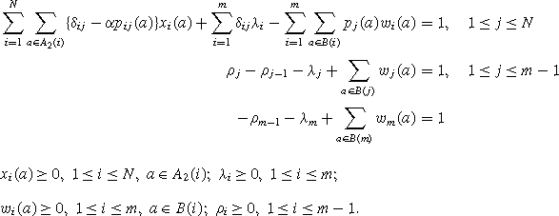Without using the transformed problem, the linear program to compute the value vector v α is:

$$\min\Biggl\{\sum_{i=1}^N v_i \Big\vert v_i \geq r_i(a)+ \alpha\sum_{j=1}^N p_{ij}(a)v_j,\ 1 \leq i \leq N, \ a \in A(i)\Biggr\}.$$
(34)

The following result can be shown.

### Lemma 8

Let the vector v be feasible for (34) and define the vector y by $$y_{i} = \max_{a \in A_{1}(i)} \{t_{a} + \alpha\sum_{j=1}^{N} p_{j}(a)v_{j}\}$$, 1≤im. Then,

1. (1)

(v,y) is a feasible solution of (32).

2. (2)

$$\sum_{i=1}^{N} v_{i} + \sum_{i=1}^{m} y_{i} \geq\sum_{i=1}^{N}v^{\alpha}_{i} + \sum_{i=1}^{m} \max_{a \in A_{1}(i)} \{t_{a} + \alpha\sum_{j=1}^{N}p_{j}(a)v^{\alpha}_{j}\}$$.

Since v α is the unique optimal solution of (34), we have shown that (v α,y α), with $$y^{\alpha}_{i} = \max_{a\in A_{1}(i)} \{t_{a} + \alpha\sum_{j=1}^{N} p_{j}(a)v^{\alpha}_{j}\}$$, 1≤im, is the unique optimal solution of (32). The next theorem shows how an optimal policy can be found from an optimal solution of problem (33).

### Theorem 23

Let (x ,λ ,w ,ρ ) be an optimal solution of (33). Define $$S_{*} = \{j\mid\sum_{a \in A_{2}(j)}x^{*}_{j}(a) > 0\}$$ and $$k_{j} = \min\{k \geq j\mid\sum_{a \in B(k)}w^{*}_{k}(a) > 0\}$$, jS\S . Take any policy $$f^{\infty}_{*} \in C(D)$$ such that $$x^{*}_{j}(f_{*}(j)) > 0$$ if jS and $$w^{*}_{k_{j}}(f_{*}(j)) > 0$$ if jS\S . Then, $$f^{\infty}_{*}$$ is well-defined and a discounted optimal policy.

#### 5.4.3 Average rewards—unichain case

Consider the problem again in the transformed model with N+m states and with zero-time and one-time transitions. This interpretation gives rise to the following linear program for the computation of the value vector ϕ.

$$\min\left\{ x \left\vert \begin{array}{l@{\quad}l}x + y_i \geq r_i(a) + \sum_{j=1}^N p_{ij}(a)y_j, & 1 \leq i \leq N,\ a \in A_2(i) \\[0.1cm]y_i \geq s_i + z_i, & 1 \leq i \leq m \\ [0.1cm]x + z_i \geq t_a + \sum_{j=1}^N p_j(a)y_j, & 1 \leq i \leq m,\ a \in B(i) \\ [0.1cm]z_i \geq z_{i+1}, & 1 \leq i \leq m - 1\end{array}\right. \right\}.$$
(35)

The dual of program (35), where the dual variables x i (a), λ i , w i (a), ρ i correspond to the four sets of constraints in (35), is:

$$\max\sum_{i=1}^N \sum_{a \in A_2(i)} r_i(a)x_i(a) + \sum_{i=1}^ms_i \lambda_i + \sum_{i=1}^m \sum_{a \in B(i)}w_i(a)$$
(36)

subject to the constraints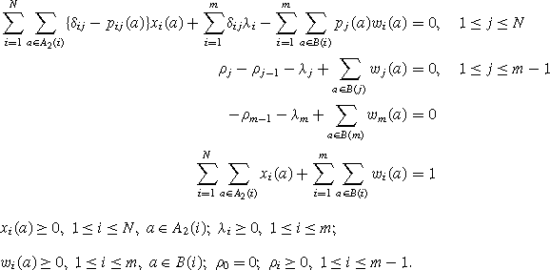Without using the transformed problem, the linear program to compute the value ϕ is:

$$\min\Biggl\{x \Big\vert x + y_i \geq r_i(a) +\sum_{j=1}^Np_{ij}(a)y_j,\ 1 \leq i \leq N,\ a \in A(i)\Biggr\}.$$
(37)

### Lemma 9

Let (x,y) feasible for problem (37) and define the vector z by $$z_{i} = \max_{a \in A_{1}(i)} \{t_{a} + \sum_{j=1}^{N}p_{j}(a)y_{j}\} - x$$, 1≤im. Then, (x,y,z) is a feasible solution of (35) and xϕ.

Since any optimal solution (x ,y ) of problem (37) satisfies x =ϕ, the optimum value of (35) is also ϕ. Furthermore, (x =ϕ,y ,z ) is an optimal solution of program (35), where $$z^{*}_{i} = \max_{a \in A_{1}(i)}\{t_{a} +\sum_{j=1}^{N} p_{j}(a)y^{*}_{j}\}-\phi$$ for i=1,2,…,m. The next theorem shows how an optimal policy can be found from an optimal solution of problem (36).

### Theorem 24

Let (x ,λ ,w ,ρ ) be an optimal solution of (36). Define $$S_{*} = \{j\mid\sum_{a \in A_{2}(j)} x^{*}_{j}(a) >0\}$$ and $$k_{j} = \min\{k \geq j\mid\sum_{a \in B(k)}w^{*}_{k}(a) > 0\}$$, $$j \in S_{w^{*}}$$, where $$S_{w^{*}} = \{j \in S \backslash S_{*}\mid \sum_{a \in A_{1}(j)} w^{*}_{j}(a) > 0\}$$. Take any policy $$f^{\infty}_{*}\in C(D)$$ such that $$x^{*}_{j}(f_{*}(j)) > 0$$ if jS , $$w^{*}_{k_{j}}(f_{*}(j)) > 0$$ if $$j \in S_{w^{*}}$$ and f (j) arbitrarily chosen if $$j \notin S_{*} \cup S_{w^{*}}$$. Then, $$f^{\infty}_{*}$$ is an average optimal policy.

#### 5.4.4 Average rewards—general case

Again, the interpretation of the transformed model gives rise to consider the following linear program in order to compute the value vector ϕ.

$$\min\left\{\sum_{j=1}^N x_j + \sum_{j=1}^m w_j\left\vert \begin{array}{l@{\quad}l}x_i \geq\sum_{j=1}^N p_{ij}(a)x_j,& 1 \leq i \leq N,\ a \in A_2(i)\\ [0.1cm]x_i \geq w_i, &1 \leq i \leq m \\ [0.1cm]w_i \geq\sum_{j=1}^N p_j(a)x_j, &1 \leq i \leq m,\ a \in B(i) \\ [0.1cm]w_i \geq w_{i+1}, &1 \leq i \leq m - 1 \\ [0.1cm]x_i + y_i \geq r_i(a) + \sum_{j=1}^N p_{ij}(a)y_j, &1 \leq i \leq N,\ a \in A_2(i) \\ [0.1cm]y_i \geq s_i + z_i, &1 \leq i \leq m \\ [0.1cm]w_i + z_i \geq t_a + \sum_{j=1}^N p_j(a)y_j,& 1 \leq i \leq m,\ a \in B(i) \\ [0.1cm]z_i \geq z_{i+1}, & 1 \leq i \leq m - 1\end{array}\right. \right\}.$$
(38)

The dual of program (38), where the dual variables y i (a), μ i , z i (a), σ i , x i (a), λ i , w i (a), ρ i correspond to the eight sets of constraints in (38), is:

$$\max\sum_{i=1}^N \sum_{a \in A_2(i)} r_i(a)x_i(a)+ \sum_{i=1}^m s_i \lambda_i + \sum_{i=1}^m \sum_{a \in B(i)}t_a w_i(a)$$
(39)

subject to the constraints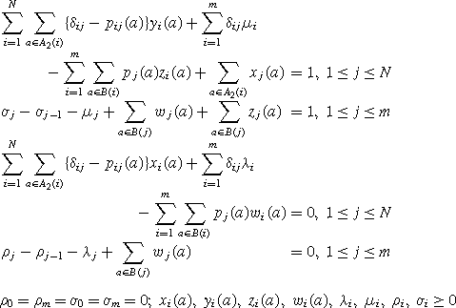for all i and a.

Without using the transformed problem, the linear program to compute the value ϕ is:

$$\min\left\{\sum_{j=1}^N x_j \left\vert \begin{array}{l@{\quad}l}\sum_{j=1}^N \{\delta_{ij} - p_{ij}(a)\} x_j \geq0, & 1 \leq i \leq N,\ a \in A(i) \\ [0.1cm]x_i + \sum_{j=1}^N \{\delta_{ij} - p_{ij}(a)\} u_j \geq r_i(a), & 1\leq i \leq N,\ a \in A(i)\end{array}\right.\right\}.$$
(40)

### Theorem 25

Let (x ,w ,y ,z ) and (y ,μ ,z ,σ ,x ,λ ,w ,ρ ) be optimal solutions of the problems (38) and (39), respectively. Let m i and n i defined by $$m_{i} = \min\{j \geq i\mid\sum_{a \in B(j)} w^{*}_{j}(a) > 0\}$$ and $$n_{i} = \min\{j \geq i\mid\sum_{a \in B(j)} \{w^{*}_{j}(a) + z^{*}_{j}(a)\} >0\}$$. Take any policy $$f^{\infty}_{*} \in C(D)$$ such that

$$\begin{array}{l@{\quad}l}x^*_i\big(f_*(i)\big) > 0& \mbox{\textit{if}}\ i \in S_*,\ \mbox{\textit{where}}\ S_* = \sum_{a \in A_2(i)} x^*_i(a) > 0; \\ [0.1cm]w^*_{m_i}\big(f_*(i)\big) > 0& \mbox{\textit{if}}\ i \notin S_*\ \mbox{\textit{and}}\ \lambda^*_i > 0; \\ [0.1cm]y^*_i\big(f_*(i)\big) > 0& \mbox{\textit{if}}\ i \notin S_*,\ \lambda^*_i = 0\ \mbox{\textit{and}}\ y^*_i\big(f_*(i)\big) > 0;\\ [0.1cm]w^*_{n_i}\big(f_*(i)\big) > 0& \mbox{\textit{if}}\ i \notin S_*,\ \lambda^*_i = \sum_{a \in A_2(i)} y^*_i(a) = 0\ \mbox{\textit{and}}\ \sum_{a \in A_1(i)} w^*_{n_i}(a) > 0; \\ [0.1cm]z^*_{n_i}\big(f_*(i)\big) > 0& \mbox{\textit{if}}\ i \notin S_*,\ \lambda^*_i = \sum_{a \in A_2(i)} y^*_i(a) = \sum_{a \in A_1(i)}w^*_{n_i}(a) = 0.\end{array}$$

Then, (1) x =ϕ; (2) $$f^{\infty}_{*}$$ is well-defined and an average optimal policy.

### Remark

De Ghellinck and Eppen (1967) have examined separable MDPs with the discounted rewards as optimality criterion. Denardo introduced in Denardo (1968) the notion of zero-time transitions. Discounted and averaging versions (for the unichain case) are then shown to yield special linear programming formulations. In the discounted case, the linear program is identical to that of De Ghellinck and Eppen. Kallenberg (1992) has shown that for the average reward criterion also in the multichain case a special linear program can be used to solve the original problem.